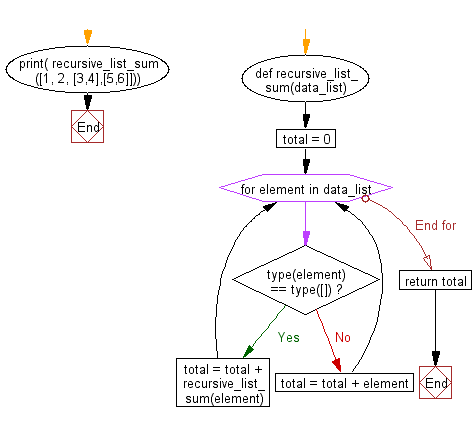﻿ Python Data Structures and Algorithms - Recursion: List sum - w3resource# Python Data Structures and Algorithms - Recursion: List sum

## Python Recursion: Exercise-3 with Solution

Write a Python program of recursion list sum.

Sample Solution:

Python Code:

``````def recursive_list_sum(data_list):
total = 0
for element in data_list:
if type(element) == type([]):
total = total + recursive_list_sum(element)
else:
total = total + element

print( recursive_list_sum([1, 2, [3,4],[5,6]]))
```
```

Sample Output:

```21
```

Flowchart:## Visualize Python code execution:

The following tool visualize what the computer is doing step-by-step as it executes the said program:

Python Code Editor:

What is the difficulty level of this exercise?

Test your Programming skills with w3resource's quiz.

﻿

## Python: Tips of the Day

Decapitalizes the first letter of a string:

Example:

```def tips_decapitalize(s, upper_rest=False):
return s[:1].lower() + (s[1:].upper() if upper_rest else s[1:])
print(tips_decapitalize('PythonTips'))
print(tips_decapitalize('PythonTips', True))
```

Output:

```pythonTips
pYTHONTIPS
```